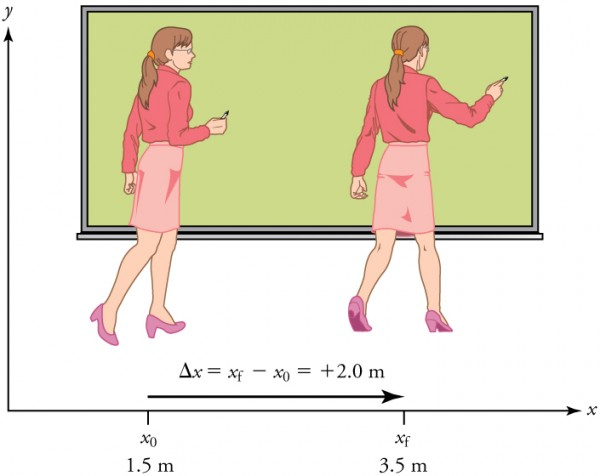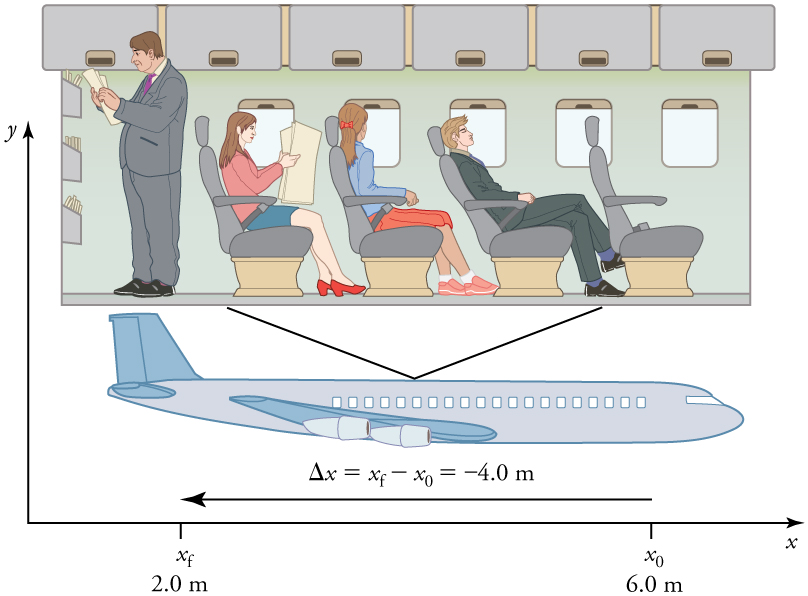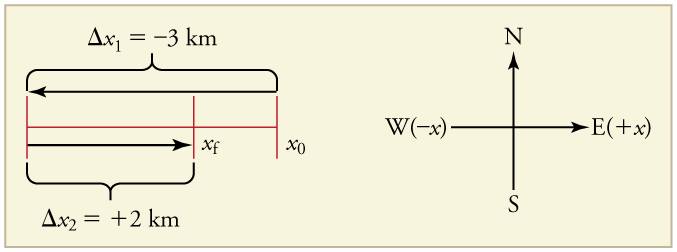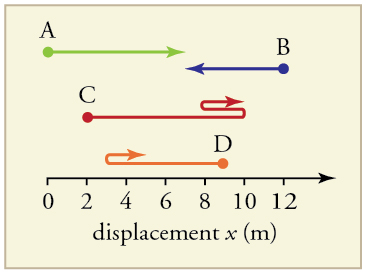## Displacement

### Learning Objectives

By the end of this section, you will be able to:

• Define position, displacement, distance, and distance traveled.
• Explain the relationship between position and displacement.
• Distinguish between displacement and distance traveled.
• Calculate displacement and distance given initial position, final position, and the path between the two.Figure 1. These cyclists in Vietnam can be described by their position relative to buildings and a canal. Their motion can be described by their change in position, or displacement, in the frame of reference. (credit: Suzan Black, Fotopedia)

## Position

In order to describe the motion of an object, you must first be able to describe its position—where it is at any particular time. More precisely, you need to specify its position relative to a convenient reference frame. Earth is often used as a reference frame, and we often describe the position of an object as it relates to stationary objects in that reference frame. For example, a rocket launch would be described in terms of the position of the rocket with respect to the Earth as a whole, while a professor’s position could be described in terms of where she is in relation to the nearby white board. (See Figure 2.) In other cases, we use reference frames that are not stationary but are in motion relative to the Earth. To describe the position of a person in an airplane, for example, we use the airplane, not the Earth, as the reference frame. (See Figure 3.)

## Displacement

If an object moves relative to a reference frame (for example, if a professor moves to the right relative to a white board or a passenger moves toward the rear of an airplane), then the object’s position changes. This change in position is known as displacement. The word “displacement” implies that an object has moved, or has been displaced.

### Displacement

Displacement is the change in position of an object:

Δx = xf − xo,

where Δx is displacement, xf is the final position, and x0 is the initial position.

In this text the upper case Greek letter Δ (delta) always means “change in” whatever quantity follows it; thus, Δx means change in position. Always solve for displacement by subtracting initial position x0 from final position xf.

Note that the SI unit for displacement is the meter (m) (see Physical Quantities and Units), but sometimes kilometers, miles, feet, and other units of length are used. Keep in mind that when units other than the meter are used in a problem, you may need to convert them into meters to complete the calculation.Figure 2. A professor paces left and right while lecturing. Her position relative to Earth is given by x. The displacement of the professor relative to Earth is represented by an arrow pointing to the right.Figure 3. A passenger moves from his seat to the back of the plane. His location relative to the airplane is given by x. The −4.0-m displacement of the passenger relative to the plane is represented by an arrow toward the rear of the plane. Notice that the arrow representing his displacement is twice as long as the arrow representing the displacement of the professor (she moves twice as far) in Figure 3.

Note that displacement has a direction as well as a magnitude. The professor’s displacement is 2.0 m to the right, and the airline passenger’s displacement is 4.0 m toward the rear. In one-dimensional motion, direction can be specified with a plus or minus sign. When you begin a problem, you should select which direction is positive (usually that will be to the right or up, but you are free to select positive as being any direction). The professor’s initial position is x0 = 1.5 m  and her final position is xf = 3.5 m. Thus her displacement is

Δx = xf − x= 3.5 m − 1.5 m = +2.0 m

In this coordinate system, motion to the right is positive, whereas motion to the left is negative. Similarly, the airplane passenger’s initial position is x0=6.0 m and his final position is xf=2.0 m, so his displacement is

Δx = xf − x= 2.0 m − 6.0 m =−4.0 m

His displacement is negative because his motion is toward the rear of the plane, or in the negative x direction in our coordinate system.

## Distance

Although displacement is described in terms of direction, distance is not. Distance is defined to be the magnitude or size of displacement between two positions. Note that the distance between two positions is not the same as the distance traveled between them. Distance traveled is the total length of the path traveled between two positions. Distance has no direction and, thus, no sign. For example, the distance the professor walks is 2.0 m. The distance the airplane passenger walks is 4.0 m.

### Misconception Alert: Distance Traveled vs. Magnitude of Displacement

It is important to note that the distance traveled, however, can be greater than the magnitude of the displacement (by magnitude, we mean just the size of the displacement without regard to its direction; that is, just a number with a unit). For example, the professor could pace back and forth many times, perhaps walking a distance of 150 m during a lecture, yet still end up only 2.0 m to the right of her starting point. In this case her displacement would be +2.0 m, the magnitude of her displacement would be 2.0 m, but the distance she traveled would be 150 m. In kinematics we nearly always deal with displacement and magnitude of displacement, and almost never with distance traveled. One way to think about this is to assume you marked the start of the motion and the end of the motion. The displacement is simply the difference in the position of the two marks and is independent of the path taken in traveling between the two marks. The distance traveled, however, is the total length of the path taken between the two marks.

A cyclist rides 3 km west and then turns around and rides 2 km east. (a) What is her displacement? (b) What distance does she ride? (c) What is the magnitude of her displacement?Figure 4.

Solutions

(a) The rider’s displacement is Δx = xf − xo=-1 km. (The displacement is negative because we take east to be positive and west to be negative.)

(b) The distance traveled is 3 km + 2 km = 5 km.

(c) The magnitude of the displacement is 1 km.

## Section Summary

• Kinematics is the study of motion without considering its causes. In this chapter, it is limited to motion along a straight line, called one-dimensional motion.
• Displacement is the change in position of an object.
• In symbols, displacement Δx is defined to be

Δx = xf − xo,

where xo is the initial position and xf is the final position. In this text, the Greek letter Δ (delta) always means “change in” whatever quantity follows it. The SI unit for displacement is the meter (m). Displacement has a direction as well as a magnitude.

• When you start a problem, assign which direction will be positive.
• Distance is the magnitude of displacement between two positions.
• Distance traveled is the total length of the path traveled between two positions.

### Conceptual Questions

1. Give an example in which there are clear distinctions among distance traveled, displacement, and magnitude of displacement. Specifically identify each quantity in your example.

2. Under what circumstances does distance traveled equal magnitude of displacement? What is the only case in which magnitude of displacement and displacement are exactly the same?

3. Bacteria move back and forth by using their flagella (structures that look like little tails). Speeds of up to 50μm/s (50 c 10-6 m/s) have been observed. The total distance traveled by a bacterium is large for its size, while its displacement is small. Why is this?

### Problems & ExercisesFigure 5.

1. Find the following for path A in Figure 5: (a) The distance traveled. (b) The magnitude of the displacement from start to finish. (c) The displacement from start to finish.

2. Find the following for path B in Figure 5: (a) The distance traveled. (b) The magnitude of the displacement from start to finish. (c) The displacement from start to finish.
3. Find the following for path C in Figure 5: (a) The distance traveled. (b) The magnitude of the displacement from start to finish. (c) The displacement from start to finish.

4. Find the following for path D in Figure 5: (a) The distance traveled. (b) The magnitude of the displacement from start to finish. (c) The displacement from start to finish.

## Glossary

kinematics:
the study of motion without considering its causes
position:
the location of an object at a particular time
displacement:
the change in position of an object
distance:
the magnitude of displacement between two positions
distance traveled:
the total length of the path traveled between two positions

### Selected Solutions to Problems & Exercises

1. (a) 7 m (b) 7 m (c)+ 7 m

3. (a) 13 m (b) 9 m (c) +9 m